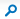# MathsShare

 This Site: MathsShareMathsShare > Puzzles > Pegs > Pegs

## Puzzles: Pegs### Body

Square peg in round hole of radius r. Cross Section Analysis: Area of Hole = (PI)rr, Length of side of peg = 2rCos45 = 2r/sqrt(2), Area of Peg = 2rr, Peg area % = 2/(PI) = 64%

Round peg of radius r in square hole. Cross Section Analysis: Area of Hole = 4rr, Area of Peg = (PI)rr, Peg area % = (PI)/4 = 79%

(rr = r squared)

In general terms a round peg in a square hole occupies more of the area of the hole than a square peg in a round hole.

### AttachmentsContent Type: Message
 Created at 21/05/2013 04:15 PM by John Rowland Last modified at 21/05/2013 04:15 PM by John Rowland